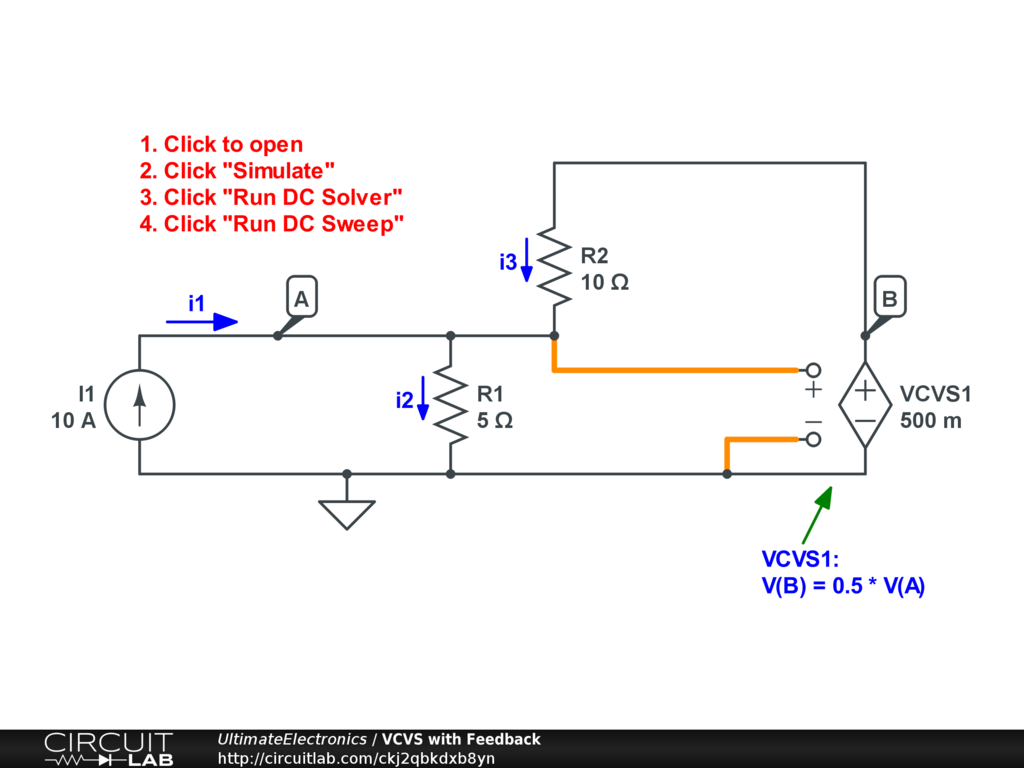Dependent Source Feedback

In the previous section, Dependent (Controlled) Sources, we considered a number of circuits where we could easily partition the input of a dependent source from its output, and solve the two halves of the circuit one at a time.

So far we've only considered cases where the controlled source's output does not interact with the controlling values. These cases are often identifiable by inspection and make solving simpler because the problem can be split in two.

However, most useful applications of dependent sources involve feedback and must be solved as a system in entirety. For example:Interactive Exercise Click to simulate the circuit above.

We can no longer analyze this with input and output separately because the output voltage is connected back to the input via resistor R2.

Instead, we can fall back on our general procedure discussed in Solving Circuit Systems to solve the circuit.

For step 1: we've labeled two non-ground nodes A and B.

For step 2: we've labeled three branch currents $i_1, i_2, i_3$ and indicated their direction with arrows.

For step 3: we can write Kirchhoff's Current Law at node A:

$$i_1 - i_2 + i_3 = 0$$

For step 4: we can write component constitutent equations for I1, R1, R2, and VCVS1:

\begin{align} i_1 & = 10 && \text{(for I1)} \\ V_A & = 5 i_2 && \text{(for R1)} \\ V_B - V_A & = 10 i_3 && \text{(for R2)} \\ V_B & = 0.5 V_A && \text{(for VCVS1)} \end{align}

For step 5: we can combine into a single system of equations with 5 equations and 5 unknowns:

\begin{align} i_1 - i_2 + i_3 & = 0\\ i_1 & = 10 \\ V_A - 5 i_2 & = 0 \\ V_B - V_A - 10 i_3 & = 0 \\ V_B - 0.5 V_A & = 0 \end{align}

or as an augmented matrix:

$$\begin{equation} \left[ \begin{array}{ccccc|c} 1 & -1 & 1 & 0 & 0 & 0 \\ 1 & 0 & 0 & 0 & 0 & 10 \\ 0 & -5 & 0 & 1 & 0 & 0 \\ 0 & 0 & -10 & -1 & 1 & 0 \\ 0 & 0 & 0 & -0.5 & 1 & 0 \\ \end{array} \right] \ \text{for} \ (i_1, i_2, i_3, V_A, V_B) \end{equation}$$

For step 6: we can solve using our process from Systems of Equations.

First, row 2 simply says $\boxed{i_1 = 10}$, so we can extract that quickly. Row 5 says $V_B = 0.5 V_A$, so we can remove $V_B$ and replace it with $0.5 V_A$ everywhere. With those two reductions, we're down to a 3x3 system:

$$\begin{equation} \left[ \begin{array}{ccc|c} -1 & 1 & 0 & -10 \\ -5 & 0 & 1 & 0 \\ 0 & -10 & -0.5 & 0 \\ \end{array} \right] \ \text{for} \ (i_2, i_3, V_A) \end{equation}$$

From here, the third row says $-10 i_3 = 0.5 V_A$, so we can remove $V_A$ and replace it with $-20 i_3$ everywhere, bringing us to a 2x2 system:

$$\begin{equation} \left[ \begin{array}{cc|c} -1 & 1 & -10 \\ -5 & -20 & 0 \\ \end{array} \right] \ \text{for} \ (i_2, i_3) \end{equation}$$

Now we can use a row operation to add $-\frac{1}{5}$ of the second row to the first row, and storing the result in place of the first row, resulting in:

$$\begin{equation} \left[ \begin{array}{cc|c} 0 & 5 & -10 \\ -5 & -20 & 0 \\ \end{array} \right] \ \text{for} \ (i_2, i_3) \end{equation}$$

The first row says $5 i_3 = -10$, or $\boxed{ i_3 = -2 }$. Substituting this into the second row we find $-5 i_2 = 20 i_3$, or $i_2 = -4 i_3$, so $\boxed{i_2 = 8}$.

Now that we have all of our currents, we can re-expand the simplifications we made earlier: $V_A = -20 i_3$ so $\boxed{V_A = 40}$, and $V_B = 0.5 V_A$ so $\boxed{V_B = 20}$.

We've now solved the full circuit:

$$\begin{equation} \begin{bmatrix} i_1 \\ i_2 \\ i_3 \\ V_A \\ V_B \end{bmatrix} = \begin{bmatrix} 10 \\ 8 \\ -2 \\ 40 \\ 20 \end{bmatrix} \end{equation}$$

Click the circuit above and click "Run DC Simulation." You'll find that these solutions by hand exactly match what the CircuitLab simulator calculates numerically.

Notice that the voltage $V_A = 20$ is a different value than it would be in other situations. For example, if we deleted the VCVS1 entirely, $V_A = 10 \cdot 5 = 50$. Or instead, if the VCVS1 became a short circuit, then $V_A = \frac {10} {5 // 10} = \frac {100} {3} \approx 33.33$. It's only with the feedback provided by VCVS1 that $V_A = 40$. You can rapidly verify these other conditions by clicking the schematic above, making these modifications, and re-running the simulation.

As an exercise: what happens if we change the VCVS's gain factor? Instead of 0.5, what happens in the neighborhood of $k=3$ (for example, compare $k=2.99$ to $k=3.01$)? Why does this happen? Try to solve by hand, or explore using the simulator (see DC Sweep simulation mode).

What's Next

In the next section, Superposition with Dependent Sources, we'll see how applying superposition and Thevenin equivalent circuits works when our circuits have dependent sources inside.